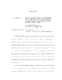## Multipath Routing Algorithms for Communication Networks: Ant Routing and Optimization Based ApproachesIn this dissertation, we study two algorithms that accomplish multipath routing in communication networks. The first algorithm that we consider belongs to the class of Ant-Based Routing Algorithms (ARA) that have been inspired by experimental observations of ant colonies. It was found that ant colonies are able to discover' the shorter of two paths to a food source by laying and following pheromone' trails. ARA algorithms proposed for communication networks employ probe packets called ant packets (analogues of ants) to collect measurements of various quantities (related to routing performance) like path delays. Using these measurements, analogues of pheromone trails are created, which then influence the routing tables. We study an ARA algorithm, proposed earlier by Bean and Costa, consisting of a delay estimation scheme and a routing probability update scheme, that updates routing probabilities based on the delay estimates. We first consider a simple scenario where data traffic entering a source node has to be routed to a destination node, with N available parallel paths between them. An ant stream also arrives at the source and samples path delays en route to the destination. We consider a stochastic model for the arrival processes and packet lengths of the streams, and a queuing model for the link delays. Using stochastic approximation methods, we show that the evolution of the link delay estimates can be closely tracked by a deterministic ODE (Ordinary Differential Equation) system. A study of the equilibrium points of the ODE enables us to obtain the equilibrium routing probabilities and the path delays. We then consider a network case, where multiple input traffic streams arriving at various sources have to be routed to a single destination. For both the N parallel paths network as well as for the general network, the vector of equilibrium routing probabilities satisfies a fixed point equation. We present various supporting simulation results. The second routing algorithm that we consider is based on an optimization approach to the routing problem. We consider a problem where multiple traffic streams entering at various source nodes have to be routed to their destinations via a network of links. We cast the problem in a multicommodity network flow optimization framework. Our cost function, which is a function of the individual link delays, is a measure of congestion in the network. Our approach is to consider the dual optimization problem, and using dual decomposition techniques we provide primal-dual algorithms that converge to the optimal routing solution. A classical interpretation of the Lagrange multipliers (drawing an analogy with electrical networks) is as potential differences' across the links. The link potential difference can be then thought of as driving the flow through the link'. Using the relationships between the link potential differences and the flows, we show that our algorithm converges to a loop-free routing solution. We then incorporate in our framework a rate control problem and address a joint rate control and routing problem.